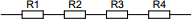# Series resistance calculator

## Calculates properties of resistors connected in series

 Example 1: Must calculate the resistance of 82kohm and 47kohm resistors connected in series View example
 Voltage volts Resistor R1 ohms Resistor R2 ohms Resistor R3 ohms Resistor R4 ohmsYou may use one of the following SI prefix after a value: p=pico, n=nano, u=micro, m=milli, k=kilo, M=mega, G=giga

 Results Total resistance 129 kilo-ohms